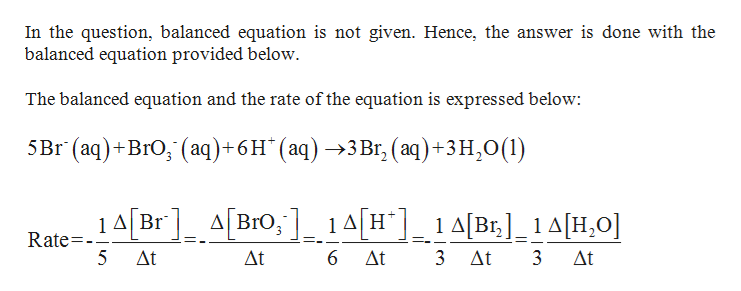# Consider the reaction4Br−(aq)+BrO−3(aq)+6H+(aq)→3Br2(aq)+3H2O(l)The average rate of consumption of Br− is 1.36×10−4 M/s over the first two minutes. What is the average rate of formation of Br2 during the same time interval?Express your answer with the appropriate units.

Question
3 views

Consider the reaction

4Br−(aq)+BrO−3(aq)+6H+(aq)→3Br2(aq)+3H2O(l)

The average rate of consumption of Br− is 1.36×10−4 M/s over the first two minutes. What is the average rate of formation of Br2 during the same time interval?

check_circle

Step 1help_outlineImage TranscriptioncloseIn the question, balanced equation is not given. Hence, the answer is done with the balanced equation provided below. The balanced equation and the rate of the equation is expressed below: 5 Br (aq)+BrO, (aq)+6H" (aq) 3Br, (aq) +3 H20(1) A[BrO,1AH14[Br]_1 A[H,0] 1ABr ABro, Rate= 3 At 5 At At 6 At 3 At fullscreen
Step 2

### Want to see the full answer?

See Solution

#### Want to see this answer and more?

Solutions are written by subject experts who are available 24/7. Questions are typically answered within 1 hour.*

See Solution
*Response times may vary by subject and question.
Tagged in

### Chemical Kinetics